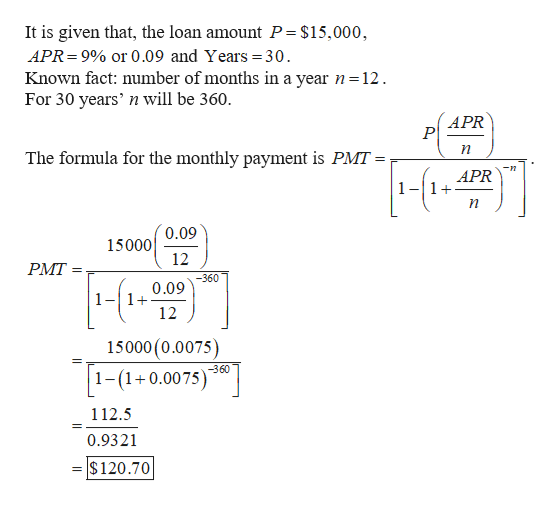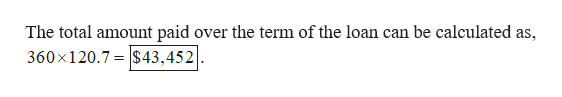# Consider a student loan of​\$15,000at a fixed APR of9​%for30years. a. Calculate the monthly payment.b. Determine the total amount paid over the term of the loan.c. Of the total amount​ paid, what percentage is paid toward the principal and what percentage is paid for interest.

Question
1 views
Consider a student loan of
​\$15,000
at a fixed APR of
9​%
for
30
years.

a. Calculate the monthly payment.
b. Determine the total amount paid over the term of the loan.
c. Of the total amount​ paid, what percentage is paid toward the principal and what percentage is paid for interest.
check_circle

Step 1

(a)

Calculate the monthly payment as follows.help_outlineImage TranscriptioncloseIt is given that, the loan amount P = \$15,000, APR 9% or 0.09 and Years 30 Known fact: number of months in a year n=12 For 30 years' n will be 360. APR P The formula for the monthly payment is PMT = APR 0.09 15000 12 PMT= -360 0.09 1-1 12 15000 (0.0075) -360 1-(10.0075 112.5 0.9321 \$120.70 = fullscreen
Step 2

(b)

Find the total amount paid ove...help_outlineImage TranscriptioncloseThe total amount paid over the term of the loan can be calculated as, 360x120.7\$43,452| fullscreen

### Want to see the full answer?

See Solution

#### Want to see this answer and more?

Solutions are written by subject experts who are available 24/7. Questions are typically answered within 1 hour.*

See Solution
*Response times may vary by subject and question.
Tagged in
MathCalculus

### Other